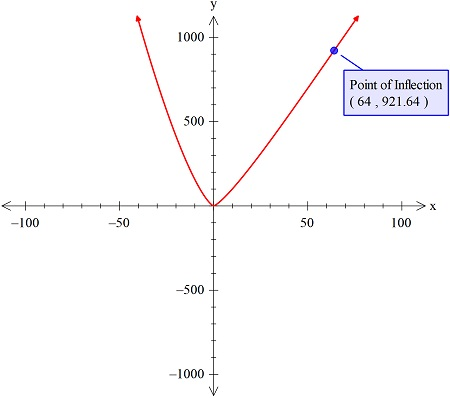# Given the function f(x) = 6^{x^{4/3}} -\frac{3}{5}^{x^{5/3}}, find the potential inflection...

## Question:

Given the function {eq}f(x) = 6x^{4/3} -\frac{3}{5}x^{5/3} {/eq}, find the potential inflection points by hand without the calculator. Use the Second Derivative Test to find the intervals on which {eq}f {/eq} is concave up or down and the inflection points.

## Concavity and Inflection Points

The concavity of the function is found with the help of the inflection point of the curve. Now, there is two methods to find the inflection point. First is the graphing utility method and second is the second derivative test method.

We will sketch the curve as follows, with the graphing utility technology.Now, we see that the inflection point is at x=64

and this will be proved using the second derivative test as follows.

Now the second derivative of the given function is:

{eq}\frac{d^2}{dx^2}\left(6x^{4/3}\:-\frac{3}{5}x^{5/3}\right)\\ =\frac{d}{dx}\left (\frac{d}{dx}\left(6x^{\frac{4}{3}}-\frac{3}{5}x^{\frac{5}{3}}\right) \right )\\ =\frac{d}{dx}\left ( \frac{d}{dx}\left(6x^{\frac{4}{3}}\right)-\frac{d}{dx}\left(\frac{3}{5}x^{\frac{5}{3}}\right) \right )\\ =\frac{d}{dx}\left(8\sqrt{x}-x^{\frac{2}{3}}\right)\\ =\frac{d}{dx}\left(8\sqrt{x}\right)-\frac{d}{dx}\left(x^{\frac{2}{3}}\right)\\ =\frac{8}{3x^{\frac{2}{3}}}-\frac{2}{3\sqrt{x}}\\ {/eq}

Now, the inflection point is when:

{eq}\frac{8}{3x^{\frac{2}{3}}}-\frac{2}{3\sqrt{x}}=0\ \Rightarrow 8-\frac{2x^{\frac{2}{3}}}{\sqrt{x}}=0\\ \Rightarrow 8-2x^{\frac{1}{3}}=0\\ \Rightarrow x^{\frac{1}{3}}=4\\ \Rightarrow x=64 {/eq}

So this is the inflection point and henc verified.

Now the curve is concave upward is when:

and concave downward when:

{eq}\frac{8}{3x^{\frac{2}{3}}}-\frac{2}{3\sqrt{x}}<0\\ \Rightarrow x>64 {/eq}